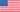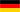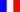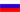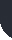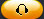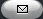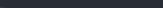Task Management Software for Team Collaboration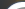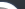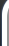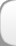HomeDownloadSupportPurchase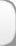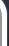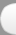Installation GuideStartUp GuideOnline Help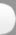# Labor Rate Variance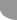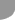Home  » Products  » VIP Task Manager Pro  » Tutorials  » Customization Guide  » Labor Rate Variance

Labor Rate Variance

Labor Rate Variance (also known as Direct Labor Rate Variance and Labor Rate Price Variance) shows the difference between Actual Labor Rate and Standard Labor Rate multiplied by the number of actual hours worked. In other words, this accounting KPI measures how much Actual Labor Rate is deviated from Standard Labor Rate for the same direct labor hours worked.

The formula to calculate Labor Rate Variance is as follows:

Labor Rate Variance = (Standard Labor Rate - Actual Labor Rate) * Direct Labor Hours

Direct Labor Hours are actual hours worked by employees.

Labor Rate Variance can be favorable or unfavorable. Standard Labor Rate should be more than Actual Labor Rate. In this case Labor Late Variance is favorable (the company underpaid its employees for direct labor and saved money). If Actual Labor Rate exceeds Standard Labor Rate, the variance is unfavorable and the company loses money by overpaying for direct labor. It can be caused by various reasons that include unreasonable Standard Labor Rate, unexpected overtime hours, shift differentials, variances in workers position and experience level, errors made by accountants.

For example, if Standard Labor Rate is \$21 USD, Actual Labor Rate is \$16.67 USD, Number of Direct Labor Hours is 3600 hours (per 2 weeks) and Labor Rate Variance is calculated as:

Labor Rate Variance = (\$21 USD  \$16.67 USD) * 3600 hours = \$4.33 USD * 3600 hours = \$15,558.00 USD

The Labor Rate Variance \$4.33 USD is favorable.

Labor Rate Variance is used to estimate how the cost of labor is close to the expected one. Labor Rate variance is indicator that shows when the company should save more money on direct labor.

• Add custom field Standard Labor Rate with Code cf_standard_labor_rate
•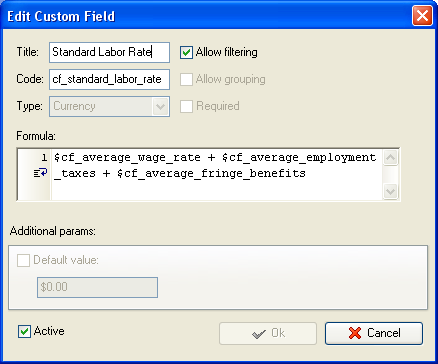• Add custom field Actual Labor Rate with Code cf_actual_labor_rate
•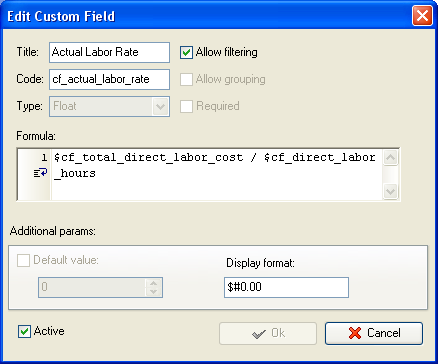• Add custom field Direct Labor Hours with Code cf_direct_labor_hours and the formula:
•
• \$cf_number_of_hours_per_day * \$cf_number_of_working_days * X * Y, where

X is the number of weeks within which you measure Labor Rate Variance

Y is the number of employees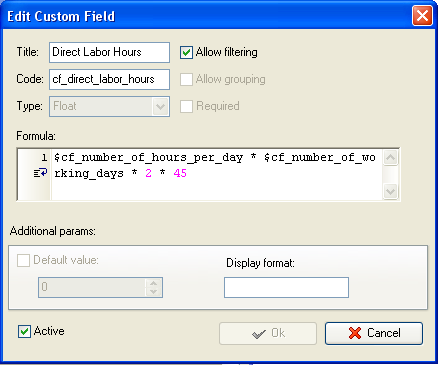• Add custom field Labor Rate Variance  with formula:
• (\$cf_standard_labor_rate - \$cf_actual_labor_rate)* \$cf_direct_labor_hours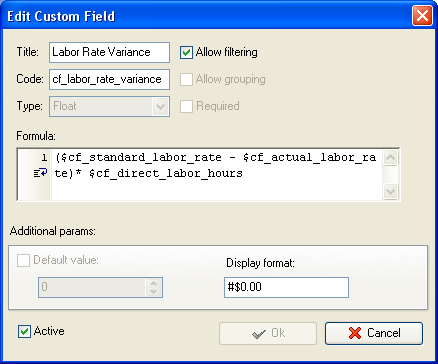• On Task Tree select task group for which you need to display Labor Rate Variance and select Custom fields options
•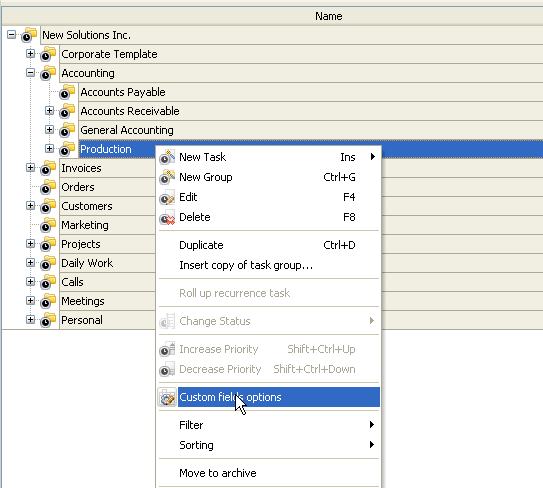• Set the following custom fields settings for the custom fields Standard Labor Rate, Actual Labor Rate, Direct Labor Hours and Labor Rate Variance:
• - In Settings for select This task group
• - select Enable
• - select Calculated by custom field formula (for task group)
•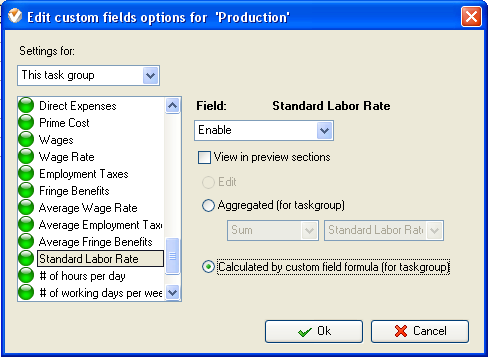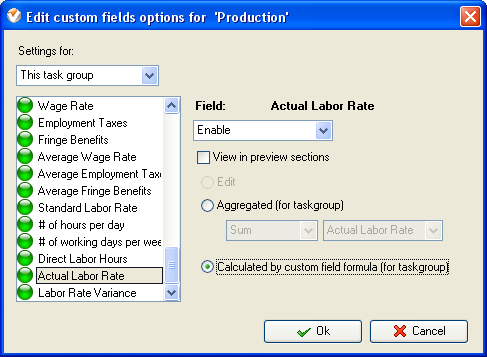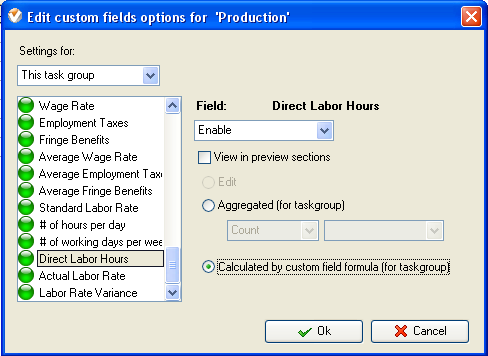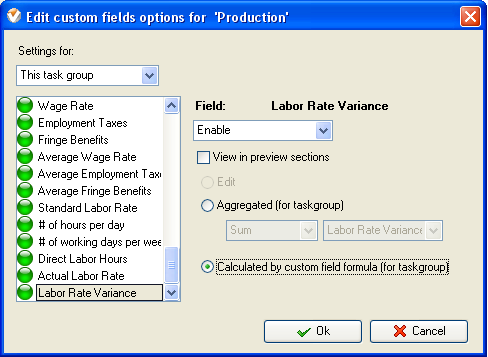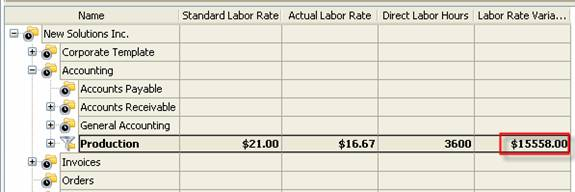Labor Rate Variance on Task Tree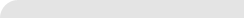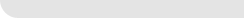» VIP Task Manager Pro VIP Task Manager Std Testimonials Turnkey Solution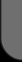ProductsSolutionsDownloadSupportPurchaseContactsForumVideoMy Account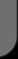Copyright © 2004 - 2023 Task Management Software